# Chapter 3 Working with factors

As what we have mentioned in the previous chapter, R sorts levels of factors in alphabetical order by default. In this chapter we will talk about working with factors using forcats package, which can be helpful when you managing categorical variables.

## 3.1 Recode factor levels

Don’t directly assign levels with `levels()<-`. Instead, using `fct_recode()`.

``````library(forcats)
x <- factor(c("G234", "G452", "G136"))
y <- fct_recode(x, Physics = "G234", Math = "G452", Chemistry = "G136")
y``````
``````##  Physics   Math      Chemistry
## Levels: Chemistry Physics Math``````

## 3.2 Relevel the factor

For the binned, ordinal data with levels out of order, `fct_relevel()` can be used to set a correct order.

``````library(tibble)
library(ggplot2)
Births2015 <- tibble(MotherAge = c("15-19 years", "20-24 years", "25-29 years", "30-34 years", "35-39 years",  "40-44 years", "45-49 years", "50 years and over", "Under 15 years"), Num = c(229.715, 850.509, 1152.311, 1094.693, 527.996, 111.848, 8.171, .754, 2.5))

ggplot(Births2015, aes(fct_relevel(MotherAge, "Under 15 years"), Num)) +
geom_col() +
coord_flip() +
scale_y_continuous(breaks = seq(0, 1250, 250)) +
ggtitle("United States Births, 2015", subtitle = "in thousands") +
theme_grey(16) +
labs(y = "mother age", x = "count")``````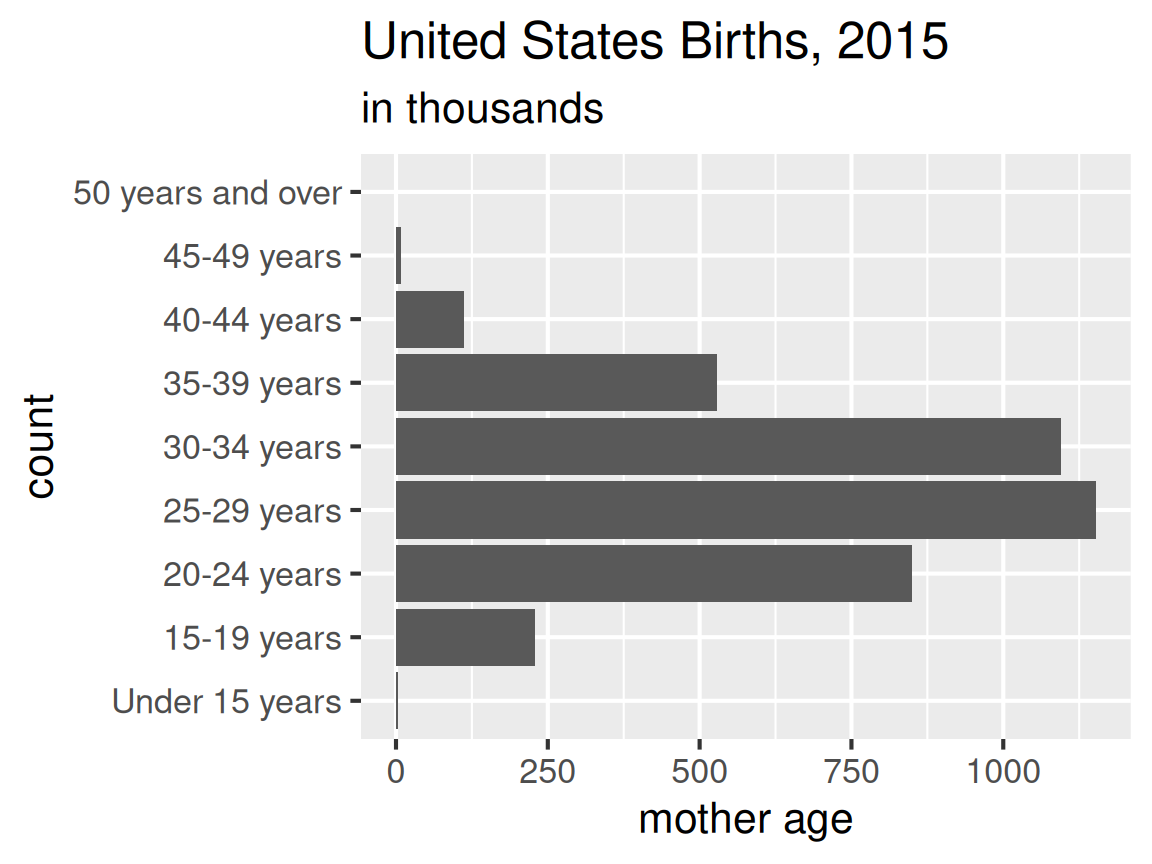The following examples give three circumstances when using `fct_relevel()`.

1. Using `fct_relevel()` to move levels to the beginning:
``````x <- c("A", "B", "C", "move1", "D", "E", "move2", "F")
fct_relevel(x, "move1", "move2")``````
``````##  A     B     C     move1 D     E     move2 F
## Levels: move1 move2 A B C D E F``````
1. Using `fct_relevel()` to move levels after an item (by position):
``````x <- c("A", "B", "C", "move1", "D", "E", "move2", "F")
fct_relevel(x, "move1", "move2", after = 4) # move after the fourth item``````
``````##  A     B     C     move1 D     E     move2 F
## Levels: A B C D move1 move2 E F``````
1. Using `fct_relevel()` to move levels to the end
``````x <- c("A", "B", "C", "move1", "D", "E", "move2", "F")
fct_relevel(x, "move1", "move2", after = Inf)``````
``````##  A     B     C     move1 D     E     move2 F
## Levels: A B C D E F move1 move2``````

If the row order is correct, use `fct_inorder()`:

``````df <- data.frame(temperature = factor(c("cold", "warm", "hot")),
count = c(15, 5, 22))

# row order is correct (think: factor in ROW order)
ggplot(df, aes(x = fct_inorder(temperature), y = count)) +
geom_col() +
theme_grey(16) +
labs( x = "temperature")``````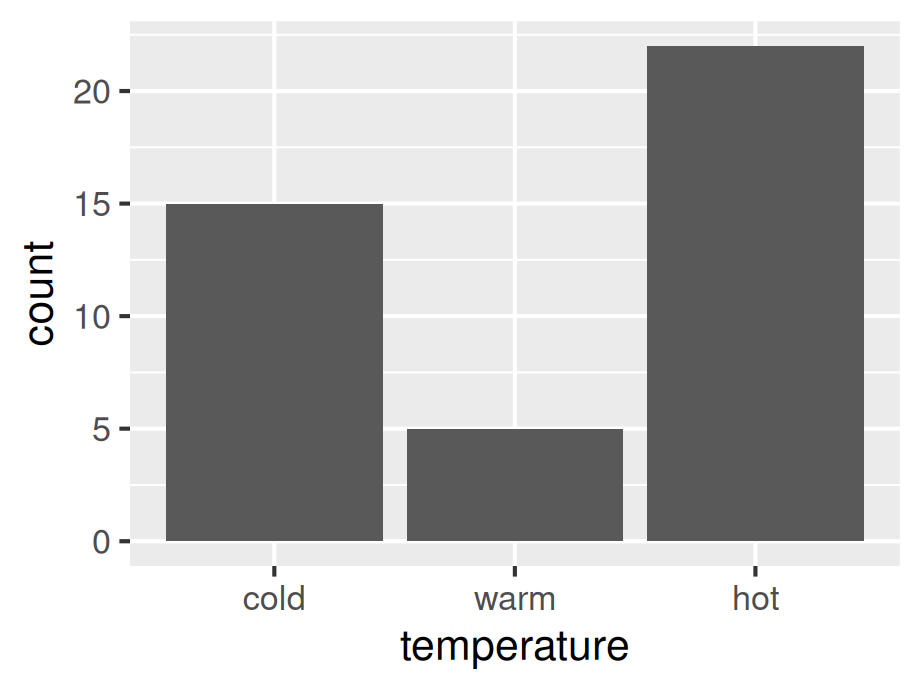## 3.3 Reorder the factors

Usually, unbinned, nominal data should be sorted by frequency order, which can be achieved using `fct_infreq()` (default is decreasing order of frequency)

``````df <- data.frame(
color = c("orange","blue", "red","brown","yellow", "green", "orange", "red", "yellow","blue","blue","red","orange","blue","red","orange","orange")
)

ggplot(df, aes(fct_infreq(color))) +
geom_bar() +
theme_grey(16)``````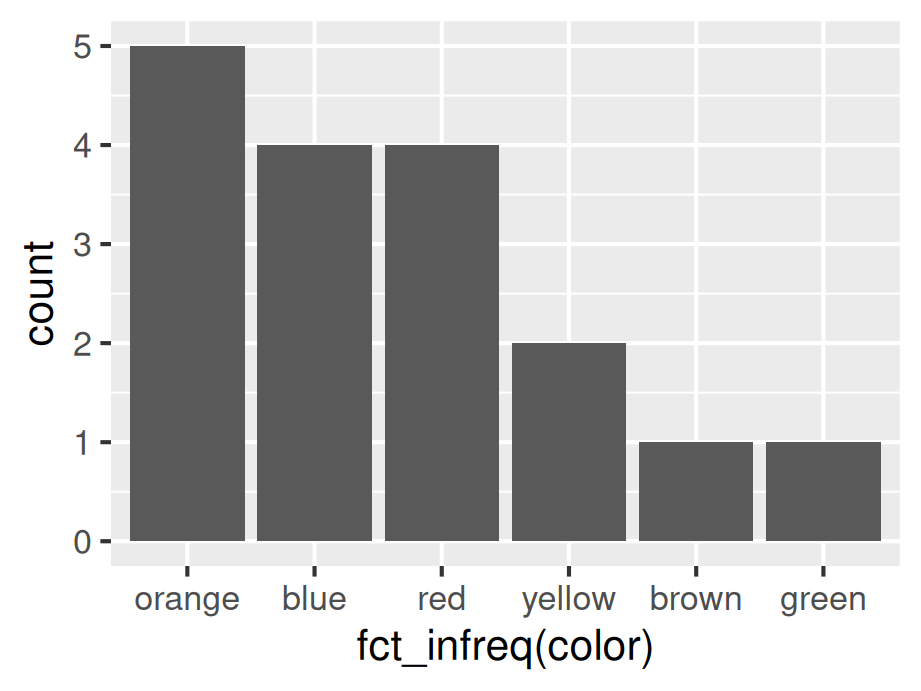For binned, nominal data which should be sorted by frequency order, use `fct_reorder()`. In the following example count is used, generally you can also apply mean,median, etc. to `.fun` inside `fct_reorder()``.

``````pack1 <- data.frame(
color = c("blue", "brown", "green", "orange", "red", "yellow"),
count = c(13, 7, 12, 9, 7, 8)
)

ggplot(pack1, aes(fct_reorder(color, count, .desc = TRUE), count)) +
geom_col() +
theme_grey(16) +
labs(x = "color")``````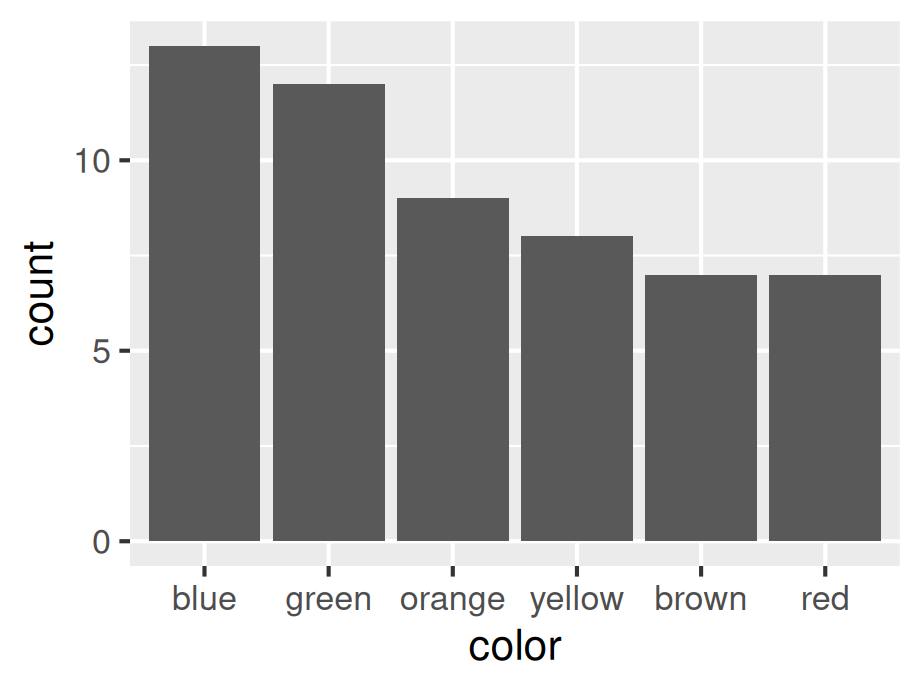## 3.4 Dealing with NAs

For prominent NA bars which should not be eliminated, use `fct_explicit_na(x)`. And using `fct_rev(x)` to reverse the factor level doesn’t help.

``````library(dplyr)
df <- data.frame(temperature = factor(c("cold", "warm", "hot", NA)), count = c(15, 5, 22, 12))

df %>%
mutate(temperature = fct_explicit_na(temperature, "NA") %>%
fct_relevel("NA", "hot", "warm", "cold")) %>%
ggplot(aes(x = temperature, y = count)) +
geom_col() +
coord_flip() +
theme_grey(16) +
labs(x = "temperature")``````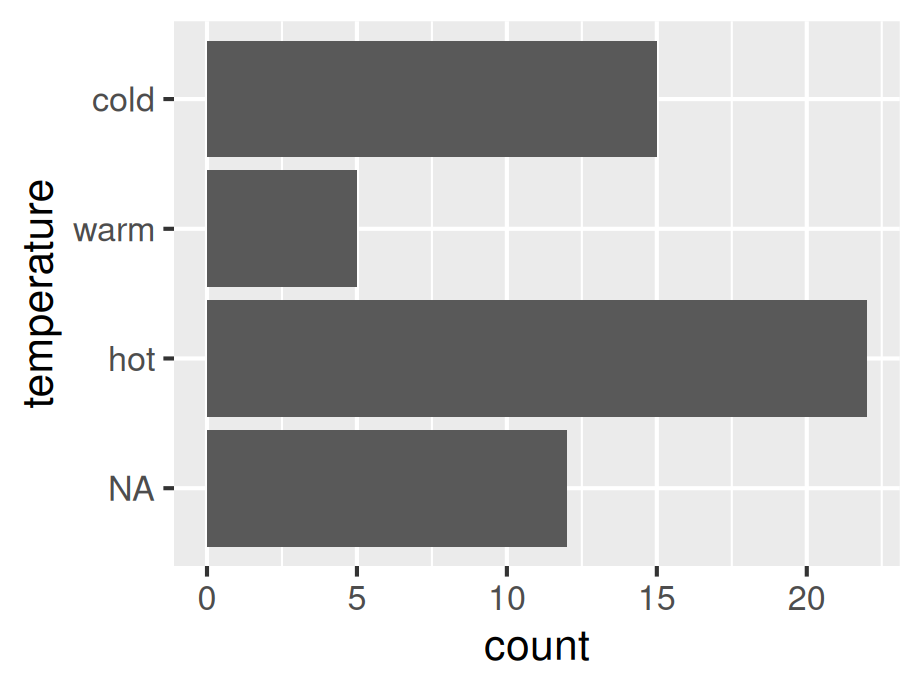## 3.5 Summary of useful functions

For analyzing categorical variables, the first step is always to decide whether the class is ordinal or nominal.

fct_recode(x, …) – change names of levels

fct_inorder(x) – set level order of x to row order

fct_relevel(x, …) – manually set the order of levels of x

fct_reorder(x, y) – reorder x by y

fct_infreq(x) – order the levels of x by decreasing frequency

fct_rev(x) – reverse the order of factor levels of x

fct_explicit_na(x) – turn NAs into a real factor level

## 3.6 Continuous to Categorical

Sometimes you want to transfer a continuous variable to a categorical variable. For example, you might want assign grades to final scores of a course. In the following example, we generated a data set of test scores randomly and we assign grades based on some thresholds. We then apply function `cut`. (You can similarly use `case_when`)

``````set.seed(2022)
testscore <- round(runif(100, min = 70, max = 100))

df <- data.frame(testscore) |>
mutate(grade = cut(testscore, breaks = seq(70, 100, 10),
labels = c("C", "B", "A"), right = FALSE,
include.lowest = TRUE))

``````##   testscore grade